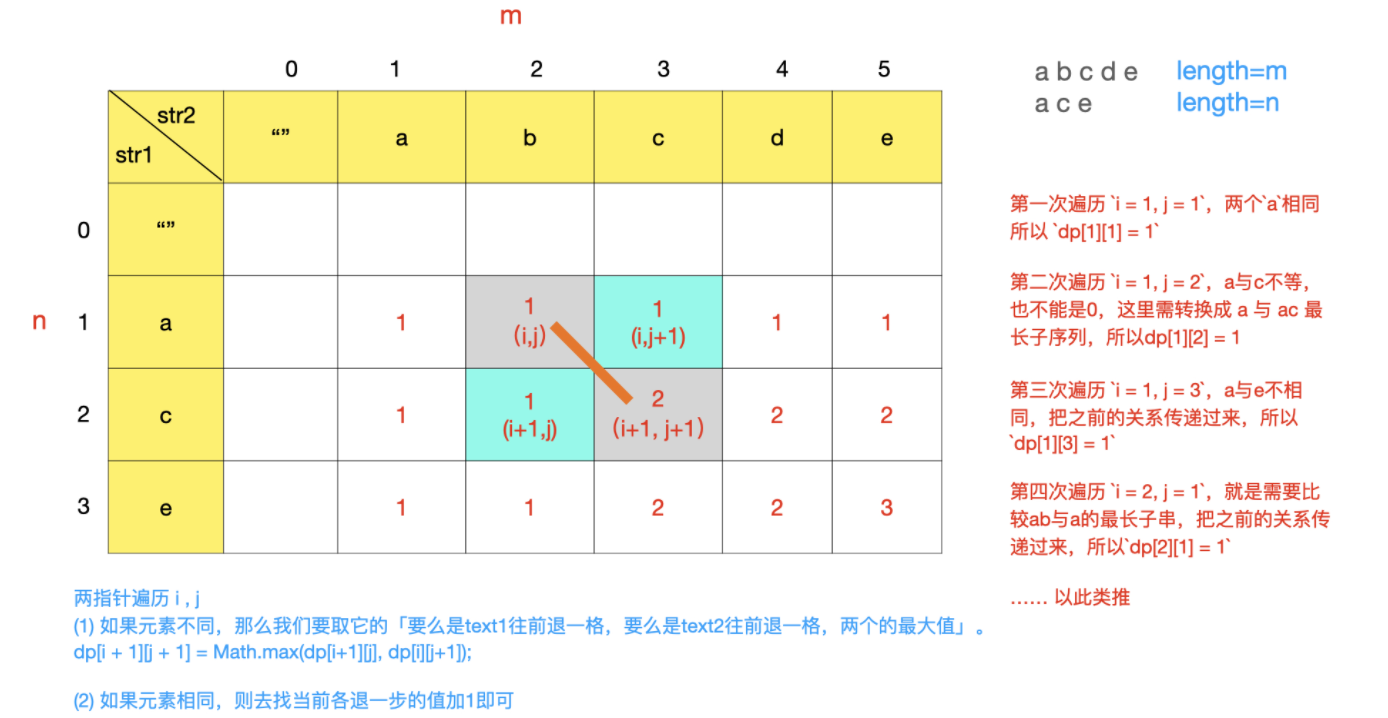# 算法攻关 - 最长公共子序列 _1143# 一、题目描述

1 <= text1.length <= 1000

1 <= text2.length <= 1000

# 二、思路text2：ace 已经走完来第一轮，接下来 text1：abcde 走到来 b 字符。

dp[i + 1][j + 1] = Math.max(dp[i+1][j], dp[i][j+1]);

# 三、代码实现

// javaclass Solution {    public int longestCommonSubsequence(String text1, String text2) {        int m = text1.length(), n = text2.length();        int[][] dp = new int[m + 1][n + 1];        for (int i = 0; i < m; i++) {            for (int j = 0; j < n; j++) {                // 获取两个串字符                char c1 = text1.charAt(i), c2 = text2.charAt(j);                if (c1 == c2) {                    // 去找它们前面各退一格的值加1即可                    dp[i + 1][j + 1] = dp[i][j] + 1;                } else {                    //要么是text1往前退一格，要么是text2往前退一格，两个的最大值                    dp[i + 1][j + 1] = Math.max(dp[i + 1][j], dp[i][j + 1]);                }            }        }        return dp[m][n];    }}

# 四、小结

DP 动态规划题目在于推导转移状态数组，感觉路很长，也是很多人不会的原因。这点需要加强## 评论